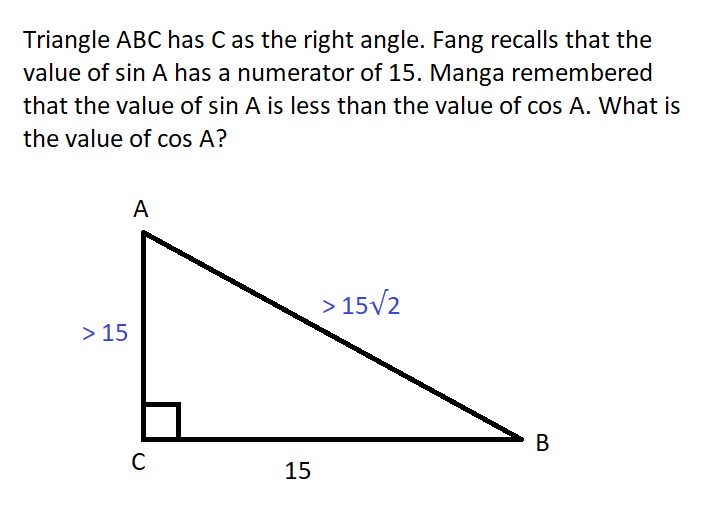# Result of the Day 3 Challenge of my May 2021 Math trigonometry mini-contest for D.Buzz 😎

in STEMGeeks2 months ago

# Problem

## Triangle ABC has C as the right angle. Fang recalls that the value of sin A has a numerator of 15. Manga remembered that the value of sin A is less than the value of cos A. What is the value of cos A?

The problem has originally been published at https://stemgeeks.net/@savvyplayer/mayday3math.

## √(2)/2 < cos A < 1 🎯

Answers which say that there is not enough information in the given will also be considered correct. 😁

# SolutionSorry for the gritty graphics, because I used MS Paint to draw the image above! 😅

Based on the given on the original problem, we have the following facts:

• C is the right angle
• sin A = 15 / BC
• sin A < cos A

Based on the facts above, we can derive the following:

• A and B are acute angles
• AC > BC, so AC > 15
• AB > 15√2 using Pythagorean theorem
• cos A = AC/AB, so cos A > √2/2
• Both AC and AB has an upper bound of positive infinity, which will approach 1, so cos A < 1

Using the last two derived facts above, we get `√2/2 < cos A < 1`.

# Winner: @minus-pi 🏅

1 HIVE has been sent to @minus-pi's Hive account. 💰